# RD Sharma Class 9 Solutions Chapter 3 Rationalisation VSAQS

## RD Sharma Class 9 Solutions Chapter 3 Rationalisation VSAQS

These Solutions are part of RD Sharma Class 9 Solutions. Here we have given RD Sharma Class 9 Solutions Chapter 3 Rationalisation VSAQS

Question 1.
Write the value of (2 + $$\sqrt { 3 }$$ ) (2 – $$\sqrt { 3 }$$).
Solution:
(2+ $$\sqrt { 3 }$$ )(2- $$\sqrt { 3 }$$ ) = (2)2-($$\sqrt { 3 }$$ )2
{∵ (a + b) (a – b) = a2 – b2}
= 4-3=1

Question 2.
Write the reciprocal of 5 + $$\sqrt { 2 }$$.
Solution: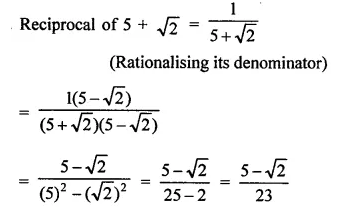Question 3.
Write the rationalisation factor of 7 – 3$$\sqrt { 5 }$$ .
Solution:
Rationalising factor of 7 – 3$$\sqrt { 5 }$$ is 7 + 3$$\sqrt { 5 }$$
{∵ ($$\sqrt { a }$$ + $$\sqrt { b }$$  ) ($$\sqrt { a }$$ – $$\sqrt { b }$$) = a-b}

Question 4.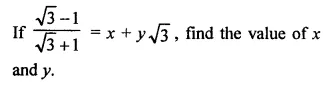Solution: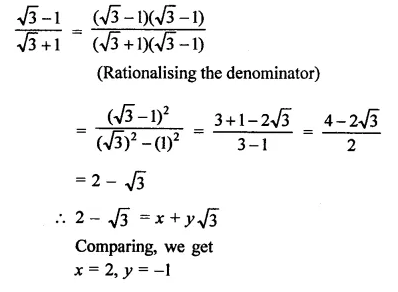Question 5.
If x =$$\sqrt { 2 }$$ – 1 then write the value of $$\frac { 1 }{ x }$$.
Solution: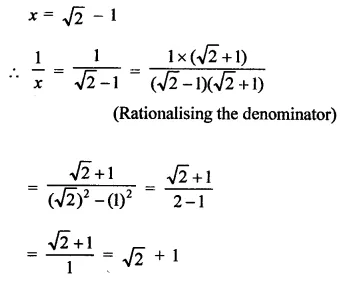Question 6.
If a = $$\sqrt { 2 }$$ + h then find the value of a –$$\frac { 1 }{ a }$$
Solution: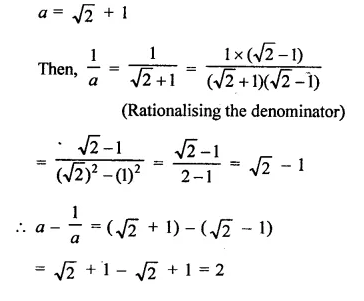Question 7.
If x = 2 + $$\sqrt { 3 }$$, find the value of x + $$\frac { 1 }{ x }$$.
Solution: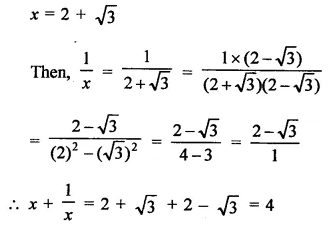Question 8.
Write the rationalisation factor of $$\sqrt { 5 }$$ – 2.
Solution:
Rationalisation factor of $$\sqrt { 5 }$$ – 2 is $$\sqrt { 5 }$$ + 2 as
($$\sqrt { a }$$ + $$\sqrt { b }$$)($$\sqrt { a }$$ – $$\sqrt { b }$$) = a – b

Question 9.
Simplify : $$\sqrt { 3+2\sqrt { 2 } }$$.
Solution: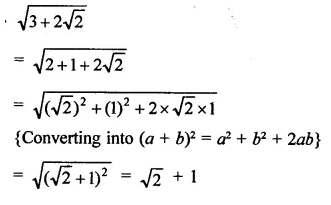Question 10.
Simplify : $$\sqrt { 3-2\sqrt { 2 } }$$.
Solution: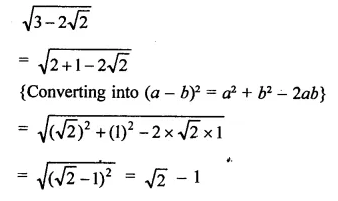Question 11.
If x = 3 + 2 $$\sqrt { 2 }$$, then find the value of  $$\sqrt { x }$$ – $$\frac { 1 }{ \sqrt { x } }$$.
Solution: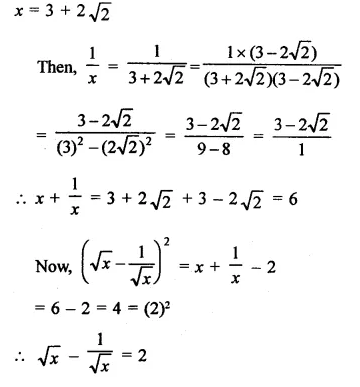Hope given RD Sharma Class 9 Solutions Chapter 3 Rationalisation VSAQS are helpful to complete your math homework.

If you have any doubts, please comment below. Learn Insta try to provide online math tutoring for you.